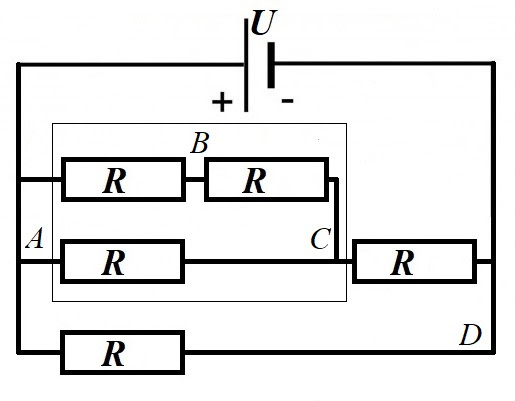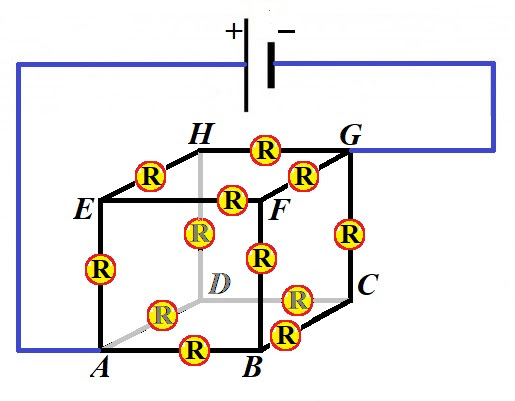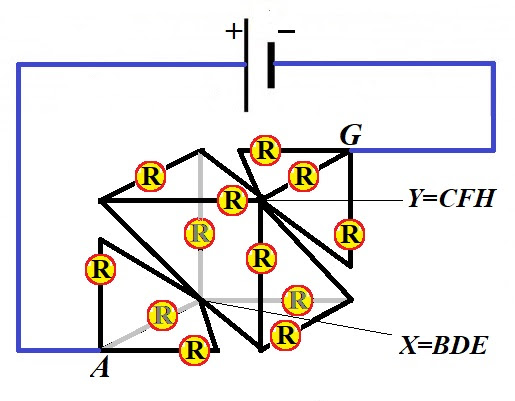## Sunday, April 5, 2020

### Unizor - Physics4Teens - Electromagnetism - DC Ohm's Law - Problems 2

Notes to a video lecture on http://www.unizor.com

Direct Current - Ohm's Law -
Problems 2

Problem A
Two resistors R1 and R2 are connected parallel to each other in a circuit.
Prove that the currents flowing through them are inversely proportional to their resistances, that is I1/I2=R2/R1.

Hint
It follows directly from the Ohm's Law.

Problem B
Given a square ABCD with diagonal AC.
Each side of this square and diagonal AC are resistors with resistance R each.
Points A and D are connected to a source of electricity with voltage U.
Determine resistance of an entire circuit RABCD and current IAC along the diagonal AC.

Solution
Redraw the circuit asand start with calculating the resistance of elements in a thin rectangle.
1/RABC = 1/R + 1/(2R) = 3/(2R)
RABC = 2R/3
Now the total resistance of a circuit is calculated as a parallel connection of the resitors with resistance (2R/3)+R=5R/3 at the top branch and another R at the bottom of a drawing
1/RABCD = [1/(5R/3)] + 1/R =
= 3/(5R) + 1/R = 8/(5R)

Total resistance of a circuit is
RABCD = 5R/8
Current from the source of electricity in the common part of a circuit is, therefore,
I = 8U/(5R)
To find the current along diagonal AC, we need to know the voltage between points A and C.
The voltage between points A and D is U.
The current in a common wire to the top branch with resistance 5R/3 is, therefore,
Itop = 3U/(5R)
The voltage in that branch drops from point A to point C by
UABC = Itop·RABC =
= 3U/(5R) · 2R/3 = 2U/5

Therefore, the current in the diagonal AC is
IAC =
RABCD = 5R/8
IAC = 2U/(5R)

Problem C
Given a cube ABCDEFGH made with identical resistors R on each edge, 12 resistors altogether.
It is connected to the source of electricity at two vertices on its main diagonal AG.What is the total resistance Rcube of this cube?

Solution
The cube is a symmetrical figure relatively to its main diagonal AG and, since all resistors are the same, one on each edge, we can use this consideration to state that the main current from the source of electricity to point A is divided into three identical currents along edges ABAD and AE.
Therefore, a voltage drop at points BD and E is exactly the same and there is no difference in electric potential between these three vertices.
If there is no difference in electric potential between points BD and E, there will be no movement of electrons between these three points if we merge these three points into one point X. The flow of electricity will not change by this merging. Analogously, the main current from the source of electricity to point G is divided into three identical currents along edges GCGF and GH.
Therefore, a voltage drop at points CF and H is exactly the same and there is no difference in electric potential between these three vertices.
If there is no difference in electric potential between points CF and H, there will be no movement of electrons between these three points if we merge these three points into one point Y. The flow of electricity will not change by this merging.
The following picture is the result of this transformation.Regardless of seemingly complex picture, it actually represents a simple circuit.
Between points A and X there are three identical parallel resistors. Their combined resistance is RAX.
RAX = R/3
Between points X and Y there are six identical parallel resistors. Their combined resistance is RXY.
RXY = R/6
Between points Y and G there are three identical parallel resistors. Their combined resistance is RYG.
RYG = R/3
These three groups are connected in a series with combined resistance
Rcube = R/3 + R/6 R/3 = 5R/6